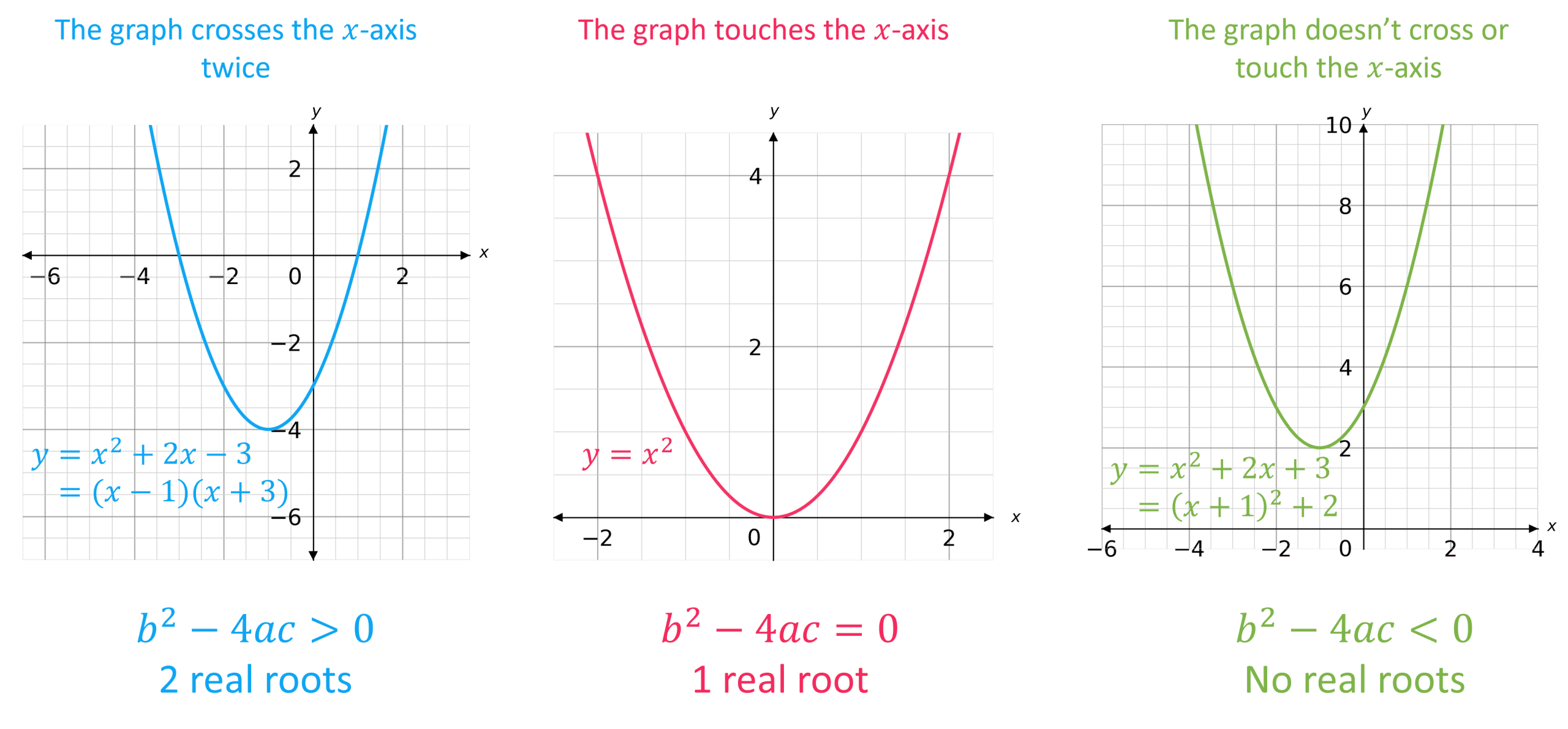A LevelAQAEdexcelOCR

The Quadratic Formula is used to find the solutions to any quadratic equation.

A LevelAQAEdexcelOCR

The solutions to the quadratic equation

$\textcolor{red}{a} x^2 + \textcolor{blue}{b}x + \textcolor{limegreen}{c} = 0$

are given by the quadratic formula:

$x=\dfrac{-\textcolor{blue}{b}\pm\sqrt{\textcolor{blue}{b}^2-4\textcolor{red}{a}\textcolor{limegreen}{c}}}{2\textcolor{red}{a}}$

Note: There are two solutions for $x$: one using $+$ and the other using $-$

A LevelAQAEdexcelOCR

## The Discriminant

The discriminant of the quadratic formula (written as $D$ or $\Delta$) is the part under the square root sign. i.e. the discriminant is

$b^2-4ac$

It can be positive, zero or negative – these tell us how many roots the quadratic equation has:

• If $b^2 - 4ac > 0$, then the quadratic has $2$ real roots (that are distinct)
• If $b^2 - 4ac = 0$, then the quadratic has $1$ real root (or ‘equal roots’)
• If $b^2 - 4ac < 0$, then the quadratic has no real roots

This can be seen visually:Note: We say no ‘real’ roots since some quadratics can have ‘imaginary’ roots – however we will not see this in this course.

A LevelAQAEdexcelOCR
A LevelAQAEdexcelOCR

## Example 1: Using the Quadratic Formula

Find the solutions to the quadratic equation $3x^2 - 8x + 2=0$, giving your answers in surd form.

[2 marks]

$a = 3$, $b = -8$ and $c = 2$

Put these values into the quadratic formula:

\begin{aligned} x &= \dfrac{-(-8) \pm \sqrt{(-8)^2 - 4 \times 3 \times 2}}{2 \times 3} \\ &= \dfrac{8 \pm \sqrt{40}}{6} \\ &= \dfrac{4 \pm \sqrt{10}}{3} \end{aligned}

So, $x = \textcolor{orange}{\dfrac{4 + \sqrt{10}}{3}}$ or $\textcolor{orange}{x = \dfrac{4 - \sqrt{10}}{3}}$

A LevelAQAEdexcelOCR

## Example 2: Finding the Discriminant

How many real roots does the quadratic equation $2x^2 - 5x + 3=0$ have?

[2 marks]

$a = 2$, $b = -5$ and $c = 3$

So, the discriminant is

$b^2-4ac = (-5)^2 - 4 \times 2 \times 3 = 1$

The discriminant is $>0$, so $2x^2 - 5x + 3=0$ has two real roots.

A LevelAQAEdexcelOCR

## Example 3: Using the Discriminant

$f(x) = 2x^2 + 4x + k$. Find the values of $k$ for which $f(x)=0$ has no real roots.

[3 marks]

$a = 2$, $b = 4$ and $c = k$

The discriminant is

$b^2 - 4ac = 4^2 - 4 \times 2 \times k = 16 - 8k$

The quadratic equation has no real roots, therefore $b^2 - 4ac < 0$

So,

\begin{aligned} 16 - 8k &< 0 \\ 16 &< 8k \\ \textcolor{orange}{k} &\textcolor{orange}{> 2} \end{aligned}

A LevelAQAEdexcelOCR

## Example 4: Using the Discriminant

$kx^2 + 2kx + 3 = 0$ has two distinct real roots.

Find the set of values for which $k$ satisfies this.

[5 marks]

$a = k$, $b = 2k$ and $c = 3$

The discriminant is

$b^2 - 4ac = (2k)^2 - (4 \times k \times 3) = 4k^2 - 12k$

Since the quadratic equation has two distinct real roots, the discriminant must be $>0$

$4k^2 - 12k > 0$

$4k^2 - 12k = 4k(k-3)$

This is $0$ when $k=0$ or when $k=3$

Hence, $4k(k-3)>0$ when $\textcolor{orange}{k<0}$ or when $\textcolor{orange}{k>3}$

Note: You can draw a graph of $y = 4k(k-3)$ to help – you would see a u-shaped graph that crosses the $k$-axis at $0$ and $3$ (see inequalities).

A LevelAQAEdexcelOCR

## The Quadratic Formula Example Questions

Rearrange the equation so that it is in the form $ax^2 + bx + c = 0$:

$2x^2 - 4x - 1 = 0$

Then, $a = 2$, $b = -4$ and $c = -1$

Put these values into the quadratic formula:

\begin{aligned} x &= \dfrac{-(-4) \pm \sqrt{(-4)^2 - 4 \times 2 \times -1}}{2 \times 2} \\ &= \dfrac{4 \pm \sqrt{24}}{4} \\ &= \dfrac{2 \pm \sqrt{6}}{2} \end{aligned}

So,

$x = \dfrac{2 + \sqrt{6}}{2} = 2.22 \text{ (2 dp)}$

$x = \dfrac{2 - \sqrt{6}}{2} = -0.22 \text{ (2 dp)}$

Rearrange the equation so that it is in the form $ax^2 + bx + c = 0$:

$-x^2 + 4x - 4 = 0$

So, $a = -1$, $b = 4$ and $c = -4$

Then, find the discriminant:

$b^2 - 4ac = 4^2 - 4(-1)(-4) = 0$

Hence, $4x - x^2 - 4 = 0$ has one real distinct root.

$a = 4$, $b=1$ and $c = 2k$

Find the discriminant:

$b^2 - 4ac = 1^2 - 4 \times 4 \times 2k = 1 - 32k$

$f(x)=0$ has two distinct real roots, therefore $b^2-4ac > 0$:

\begin{aligned} 1 - 32k &> 0 \\ 1 &> 32k \\ k &< \dfrac{1}{32} \end{aligned}

a) $a = (m+1)$, $b =(m+1)$ and $c = 2$

Find the discriminant:

\begin{aligned} b^2 - 4ac &= (m+1)^2 - 4 \times (m+1) \times 2 \\ &= (m^2 + 2m + 1) - (8m + 8) \\ &= m^2 -6m - 7 \end{aligned}

The equation has two real distinct solutions, therefore $b^2 - 4ac >0$:

$m^2 -6m - 7 > 0$

b) $m^2 -6m - 7 = (m-7)(m+1)$

This expression is $0$ when $k = 7$ and $k = -1$

Hence, $(m-7)(m+1)>0$ when $m< -1$ or when $m>7$

A Level

A Level

## You May Also Like...### MME Learning Portal

Online exams, practice questions and revision videos for every GCSE level 9-1 topic! No fees, no trial period, just totally free access to the UK’s best GCSE maths revision platform.

£0.00

A Level